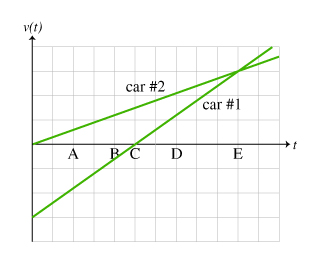# Problem: Two cars travel on the parallel lanes of a two-lane road. The cars are at the same location at time t=0s, and move in such a way as to produce the velocity (relative to the ground) vs. time graph shown in the figure. (Figure 1)On the graph, one vertical block is equivalent to one velocity unit.Rank the distance between the cars at the lettered times.Rank from largest to smallest. To rank items as equivalent, overlap them.

###### FREE Expert Solution

Uniform accelerated motion (UAM) equations:

Assume 1 horizontal block is 1s.

At A:

• vf,1 = 0.5
• v0,1 = 0
• vf,2 = -1.75
• v0,2 = -3
• Δt = 2 - 0 = 2

Δx2 - Δx1 = [(0.5 + 0)/2 × 2] - [(-1.75 + (-3))/2 ×2] = 5.25

100% (44 ratings)###### Problem Details

Two cars travel on the parallel lanes of a two-lane road. The cars are at the same location at time t=0s, and move in such a way as to produce the velocity (relative to the ground) vs. time graph shown in the figure. (Figure 1)On the graph, one vertical block is equivalent to one velocity unit.Rank the distance between the cars at the lettered times.

Rank from largest to smallest. To rank items as equivalent, overlap them.